# Tractive Effort

Definition: Tractive effort is defined as the force at the rims or the outer edges of the driving wheels of moving trains. In other words, it is the sum of the tractive force and rolling effort on the road surface. In the main line train, tractive effort is caused by the locomotive, and in a suburban train, it is caused by motor coaches.The draw pull bar force is the horizontal force available for the vehicles for pulling the load. This force is less than the tractive effort required to move the locomotive. The maximum allowable tractive effort that can be applied without wheel slip is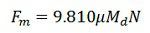Where μ is the coefficient of adhesion and Md the adhesive weight or weight on the driving wheel.

## Functions of the Tractive effort

The following are the functions perform by the tractive effort on the vehicles.

1. Tractive effort required to accelerate the train mass horizontally (in newtons) at an acceleration of α isWhere M is the mass in tonnes

2. The tractive effort required to accelerate the rotating parts: The rotating parts consist of wheels, gears, axles and rotor of the motor. The moment of inertia of the wheel is expressed by the formula shown below.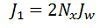Where Jw is the moment of inertia of the wheel, kgm2 and Nx is the number of axles on the wheel.

N – the number of driving motor.
n1 – teeth on motor gear wheel
n2 – teeth on axle gear wheelR – radius of the wheel, m
Jm – moment of inertia of one motor, kg-m2

Then moment of inertia of motor referred to wheelsTraction effort for driving rotating parts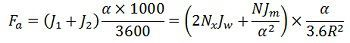Total tractive effect required for accelerating the train on a level track.Where Me is the effective mass of the train.The above equation can also be written as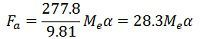3. The tractive effort required to overcome the force due to gravity: When moving up in the slope the drive has to produce tractive effort to overcome the force due to gravity.In railway, the gradient or slope is expressed as a rise in meters in a track distance of 1000 m and is denoted by G.The tractive force required to overcome the force due to gravity will be4.The tractive effort required to overcome train resistance: The resistance of the train is mainly due to various kinds of friction. The three basic types of friction responsible for the train resistances are Coulomb friction, viscous friction and air friction.

The Coulomb friction is produced by the relative motion of the two surfaces. It does not depend on the speed of the train. The viscous friction is directly proportional to the speed of the train, and the air friction is independent of the speed square.Where V is the speed of the train, and A, B, C are constants.

5. The total tractive effort required to move the train:The positive sign is used for the train movement up-gradient and negative for down gradient.

6. Motor Torque Rating:

Total torque at the rim of the driving wheels = Total tractive effort X Rwhere R is the radius of the driving wheels in meters.Total torque referred to the motor shaft is expressed by the equation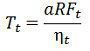where ηt is the efficiency of transmission.

Torque per motorwhere N is the number of motors

When deciding motor rating, maximum gradient allowed while laying out down the track should be considered.